# Electrical properties

## Ⅰ. Dielectric breakdown strength

Figure 28 shows the sample thickness dependence and Figure 29 shows the water-absorption dependence of dielectric breakdown strength in nylon 6.

•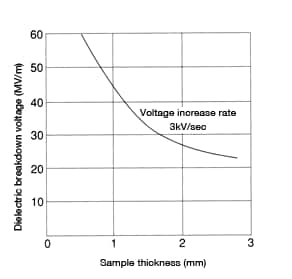Figure 28: Sample thickness dependence of dielectric breakdown voltage in nylon 6
•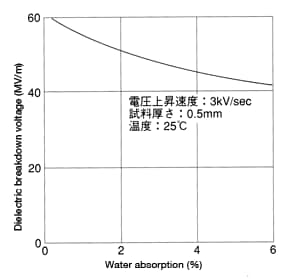Figure 29: Water-absorption dependence of dielectric breakdown voltage in nylon 6 (in a dry state at 25°C)

## Ⅱ. Dielectric properties

In a dry state, dielectric constant ε can be found with the following equation if the frequency falls between 50kHz and 20MHz.

ε = a log f + {b (f: Frequency (Hz))

Constants a and b vary depending on the type of nylon, as shown in Table 2.

Table 2: Values for dielectric constants a and b
Type of nylon a b
CM1017 (non-reinforced nylon 6) -0.15 4.25
CM2001 (non-reinforced nylon 610) -0.10 3.75
CM3001-N (non-reinforced nylon 66) -0.20 3.80

The dielectric constant and dielectric tangent change with water absorption. That effect is amplified at lower frequencies. Figure 30 shows the change over time in the dielectric constant of CM1017 (nylon 6) immersed in 20°C water. Figure 31 shows the change in the dielectric tangent of CM1017 (nylon 6) immersed in 20°C water.

•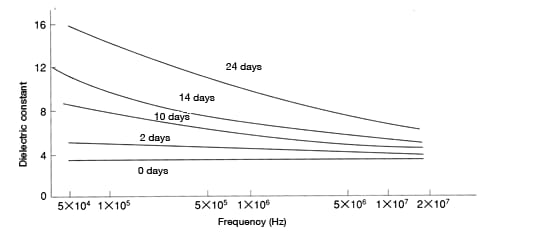Figure 30: Change over time in the dielectric constant of CM1017 (nylon 6) immersed in 20°C water

•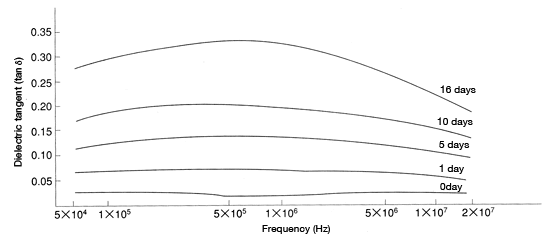Figure 31: Change over time in the dielectric tangent of CM1017 (nylon 6) immersed in 20°C water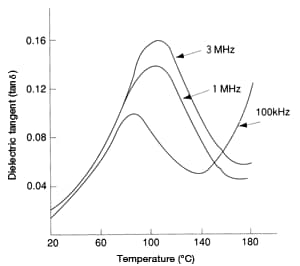Figure 32: Change in dielectric tangent of
CM3001-N (nylon 66) as a function of
temperature (in a dry state)

Figure 32 shows the temperature dependence of the dielectric tangent of CM3001-N (nylon 66)
The nylons exhibit no pronounced difference in a dry state. However, water absorption causes disparities in the properties of the different nylon grades. Therefore, used in an electrical component, CM3001-N (nylon 66) exhibits more advantages than CM1017 (nylon 6). That said, there is little difference among the different types of nylon at frequencies above 10MHz, where the effects of water absorption are negligible.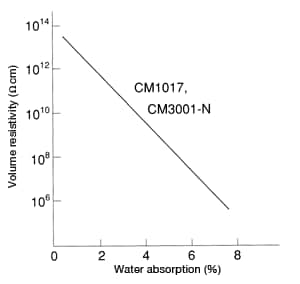Figure 33: Change in volume resistivity of
CM1017 and CM3001-N as a function of
water absorption

## Ⅲ. Volume resistivity

Figure 31 shows the water-absorption dependence of volume resistivity in CM1017 (nylon 6) and CM3001-N (nylon 66). Note that a 1% increase in water absorption results in a single-digit decrease in volume resistivity.